### Astrophysics

We have seen that all matter emits light constantly in the form of thermal radiation. In this quiz, we will take a step further and begin to understand why matter emits EM radiation as we examine the forces within individual atoms.

In addition to gravity, matter particles are subject to another long-range force that is crucial to understanding astrophysical phenomena. The electromagnetic force binding atoms together endows each kind of atom with a unique structure that is reflected in its emission spectrum.If you have ever seen Aurora Borealis (the northern lights) or Aurora Australis (the southern lights) you have directly observed a gas emission spectrum. Emission spectra are useful to astronomers because their wavelengths are uniquely related to the electronic structure of atoms. We will begin to understand this connection by pulling on the single electron in the simplest kind of atom, hydrogen.

# Atomic Spectra

Protons and electrons, subatomic particles within common matter, carry electric charge. Thus, the behavior of atoms is dominated by electric forces. The electric force law, known as Coulomb's law, is strikingly similar to gravity.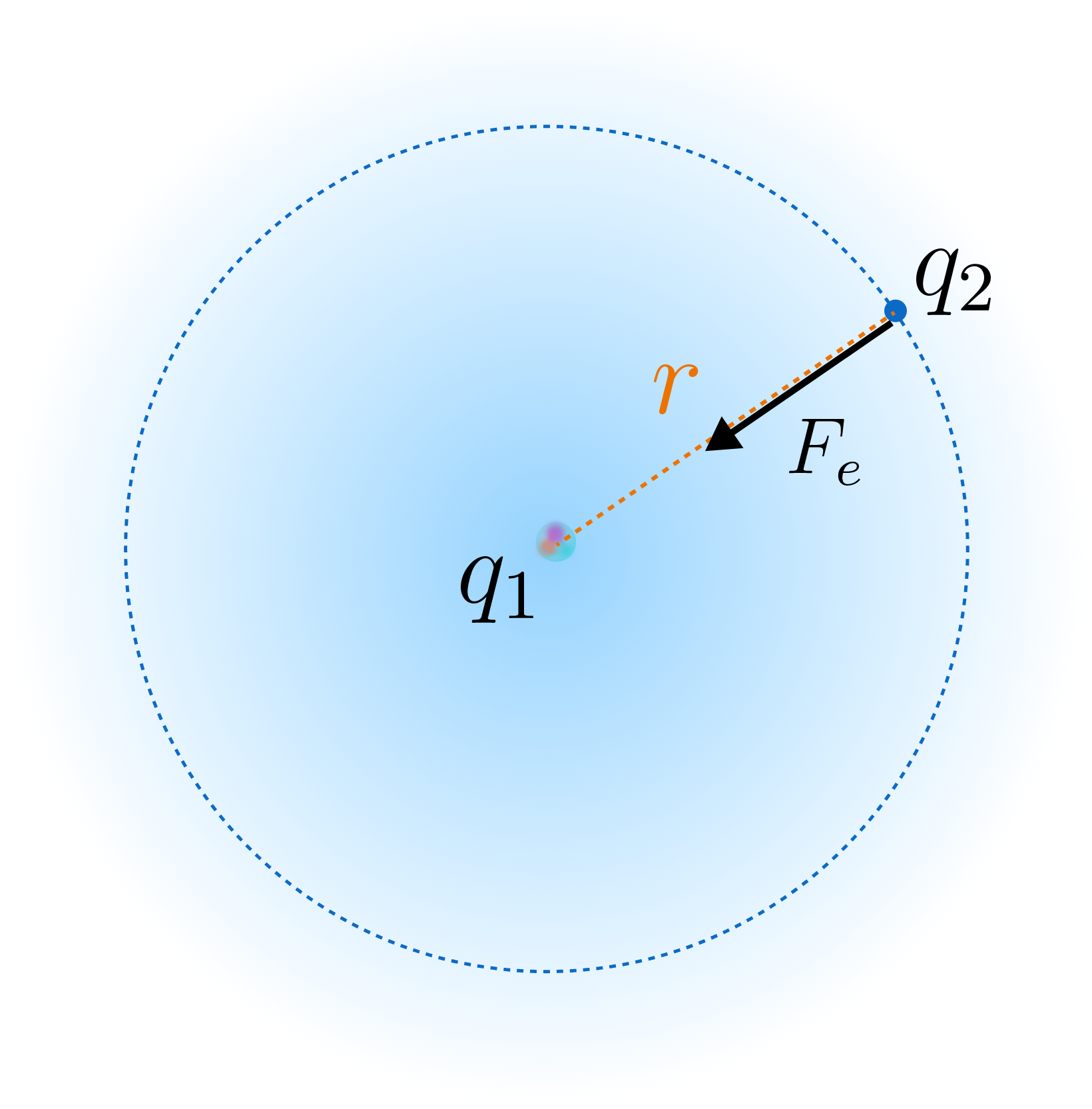Two charges $q_1$ and $q_2$ separated by a distance $r$ each feel an electric force $F_e$ according to $F_e=\frac{K_e q_1 q_2}{r^2},$ where $K_e=\SI[per-mode=symbol]{8.99e9}{\joule\meter\per\coulomb\squared}.$

In a hydrogen atom, the electron is measured at a radius of $r_B=\SI{5.29e-11}{\meter}.$ What is the force on the electron?


Details and Assumptions:

• Both the electron and the proton carry the elementary charge $q_e=\SI{1.60e-19}{\coulomb}.$ This is the basic unit of charge in our universe.

# Atomic Spectra

Quantum mechanics, the set of rules governing subatomic particles, alters the behavior of an electron in an atom. Despite the electric force's similarity to gravity, electrons do not orbit the nucleus the way planets orbit the Sun.

In fact, one of the central results of quantum mechanics is that electrons do not have a definite position (until they are measured), only a definite energy. Thus, atomic physics is framed around energy, not forces and accelerations.

By analogy with the gravitational force, the potential energy between two charges is $U_e = \frac{K_e q_1 q_2}r,$ where $K_e=\SI[per-mode=symbol]{8.99 e 9}{\joule\meter\per\coulomb\squared}.$

What is the potential energy of an electron measured at a radius of $r_B=\SI{5.29e-11}{\meter}?$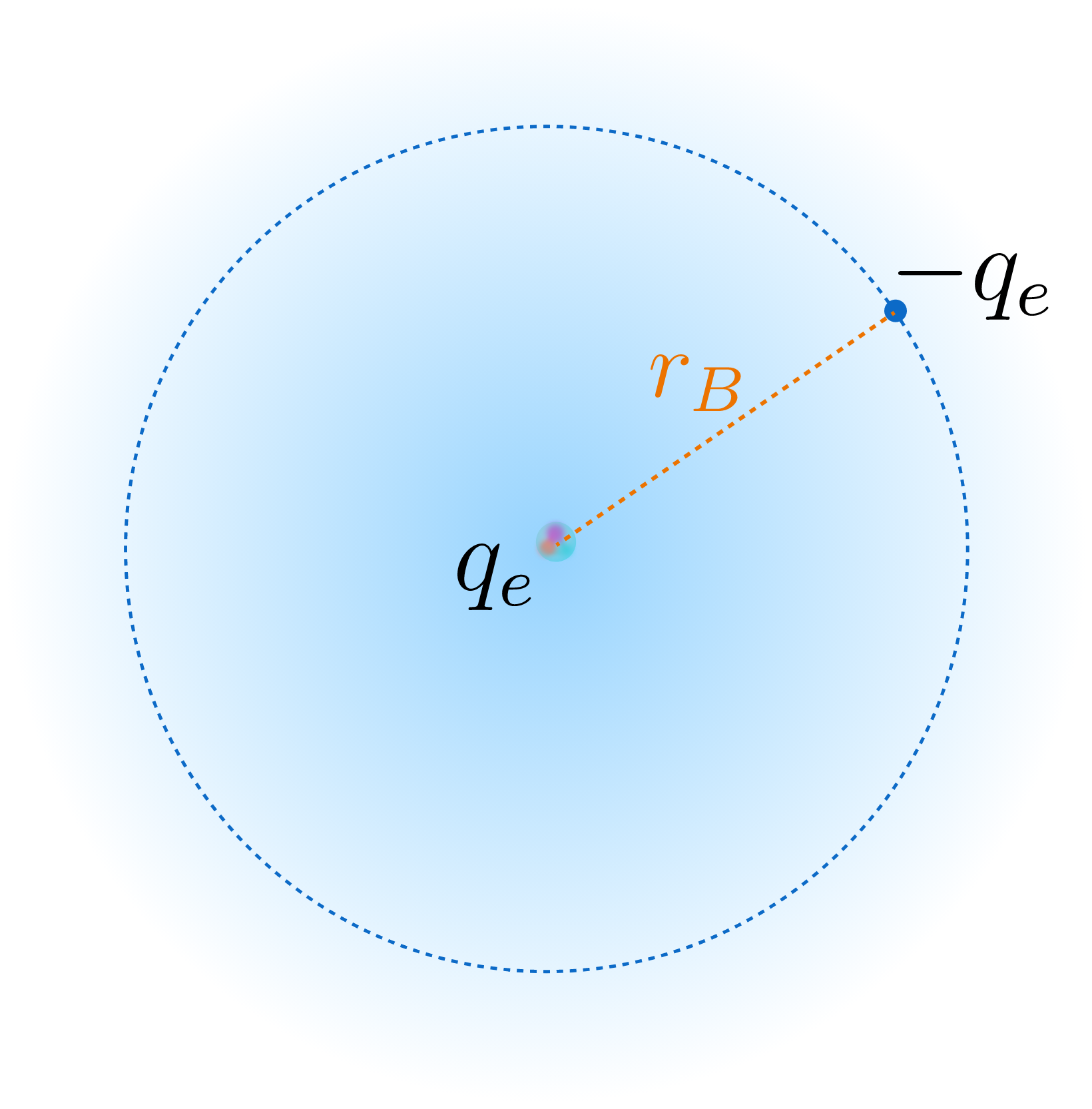Details and Assumptions:

• Both the electron and the proton carry the elementary charge $q_e=\SI{1.60e-19}{\coulomb}.$
• Like gravity, a body attracted to another has negative potential energy until it escapes.

# Atomic Spectra

In the gravity quiz, we noticed that the kinetic energy $K$ of an object in orbit obeyed a simple relationship with its potential energy $U$. Namely, $K=-\frac U2.$ This means that the total energy of an object within the Sun's gravitational influence $(K+U)$ is
$E_\text{tot}=-\frac12 U+U=\frac12 U.$ This is called the virial theorem, and it applies also to electrons and atomic nuclei bound by electric forces.Apply the virial theorem to the result of the previous question to estimate the total energy of an electron orbiting a hydrogen nucleus.


Details and Assumptions:

• Express your answer in electron-volts $(\si{\electronvolt})$ instead of Joules. $\SI{1}{\electronvolt}=\SI{1.60e-19}{\joule}.$ To avoid working with extremely small quantities, as in the previous question, electron-volts are employed when describing atomic processes.

# Atomic Spectra

Electrons in hydrogen and other atoms occupy a set of orbitals that have definite energies. In the last question, you found the ground state energy of hydrogen—the lowest energy orbital. Each orbital has a distinct shape corresponding to where the electron is more likely to be measured.One of Hydrogen's electron orbitals. The first few orbital energies are in the table below. $\begin{array}{c|c} \hline \text{Orbital}& \text{Energy} \\ \hline \text{Ground}& \SI{-13.6}{\electronvolt} \\ \hline 1^\text{st} \text{ excited}& \SI{-3.4}{\electronvolt} \\ \hline 2^\text{nd} \text{ excited}& \SI{-1.5}{\electronvolt} \\ \hline 3^\text{rd} \text{ excited}& \SI{-0.85}{\electronvolt} \\ \hline 4^\text{th} \text{ excited}& \SI{-0.54}{\electronvolt} \\ \hline \end{array}$ The important thing to remember about electrons is, at any time, they take one of a specific set of energies that are stacked within the atom. For our purposes, we do not need to understand the exact way these orbital energies are calculated using quantum mechanics in order to understand how light is emitted in Aurora Borealis or by gasses in a stellar atmosphere

# Atomic Spectra

An electron does not have to remain in the same orbital forever. Radiation passing by can be absorbed by an electron, which boosts it into a higher-energy orbital (called an excited state).

If you measured the average position and average velocity of the electron in this higher-energy state, what would you measure?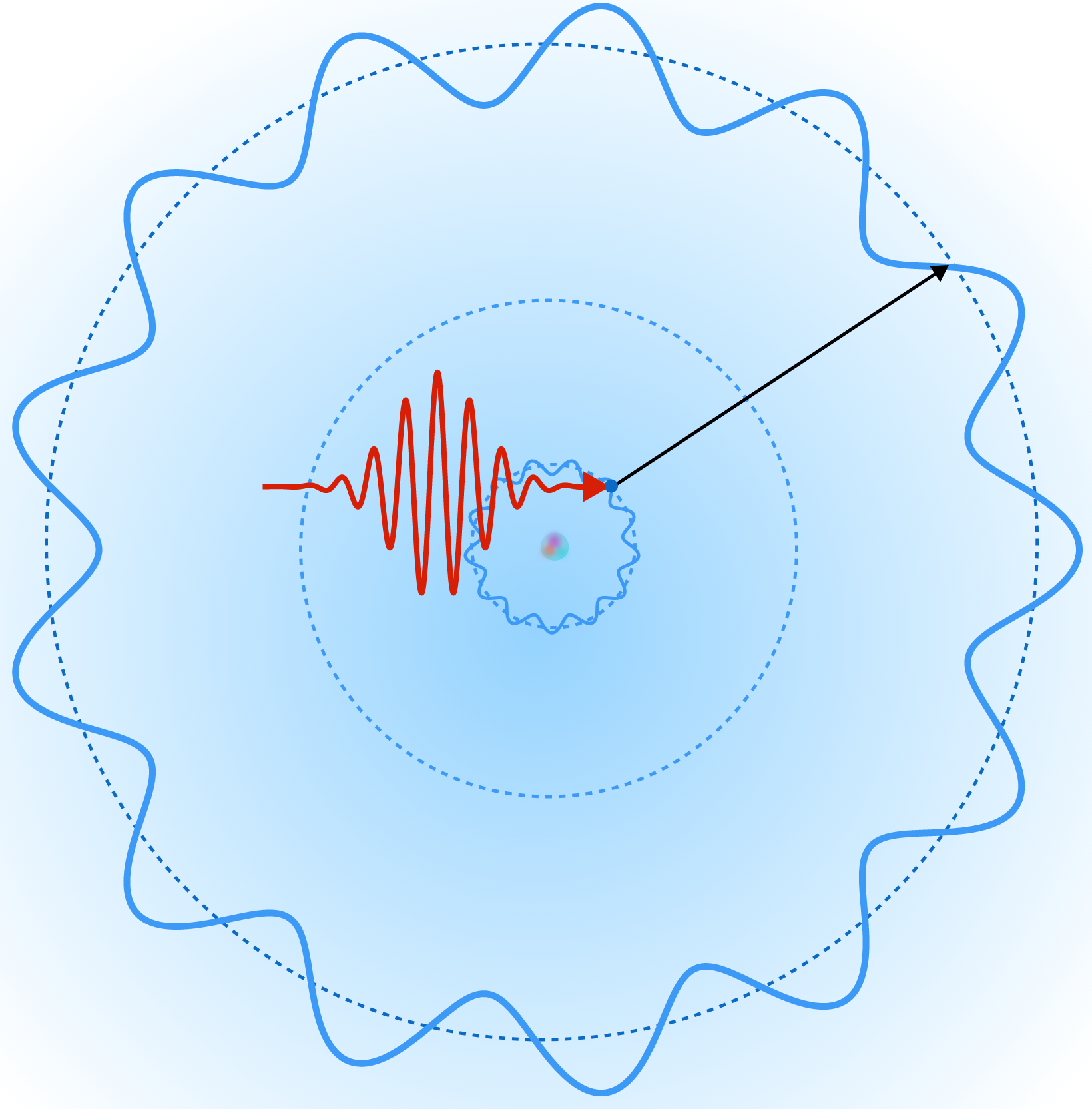Details and Assumptions:

• Electron orbitals have complicated shapes. For simplicity, we visualize the energy levels as wavy classical orbits, but keep in mind that the electron is actually smeared over a three-dimensional orbital.

# Atomic Spectra

Excited electron states are not stable. Like a rock on a hill, the electron can "fall" back to a lower-energy state. Conservation of energy says when the electron loses energy, something else must gain energy. Consequently, the electron emits a single photon as it transitions to a lower-energy state.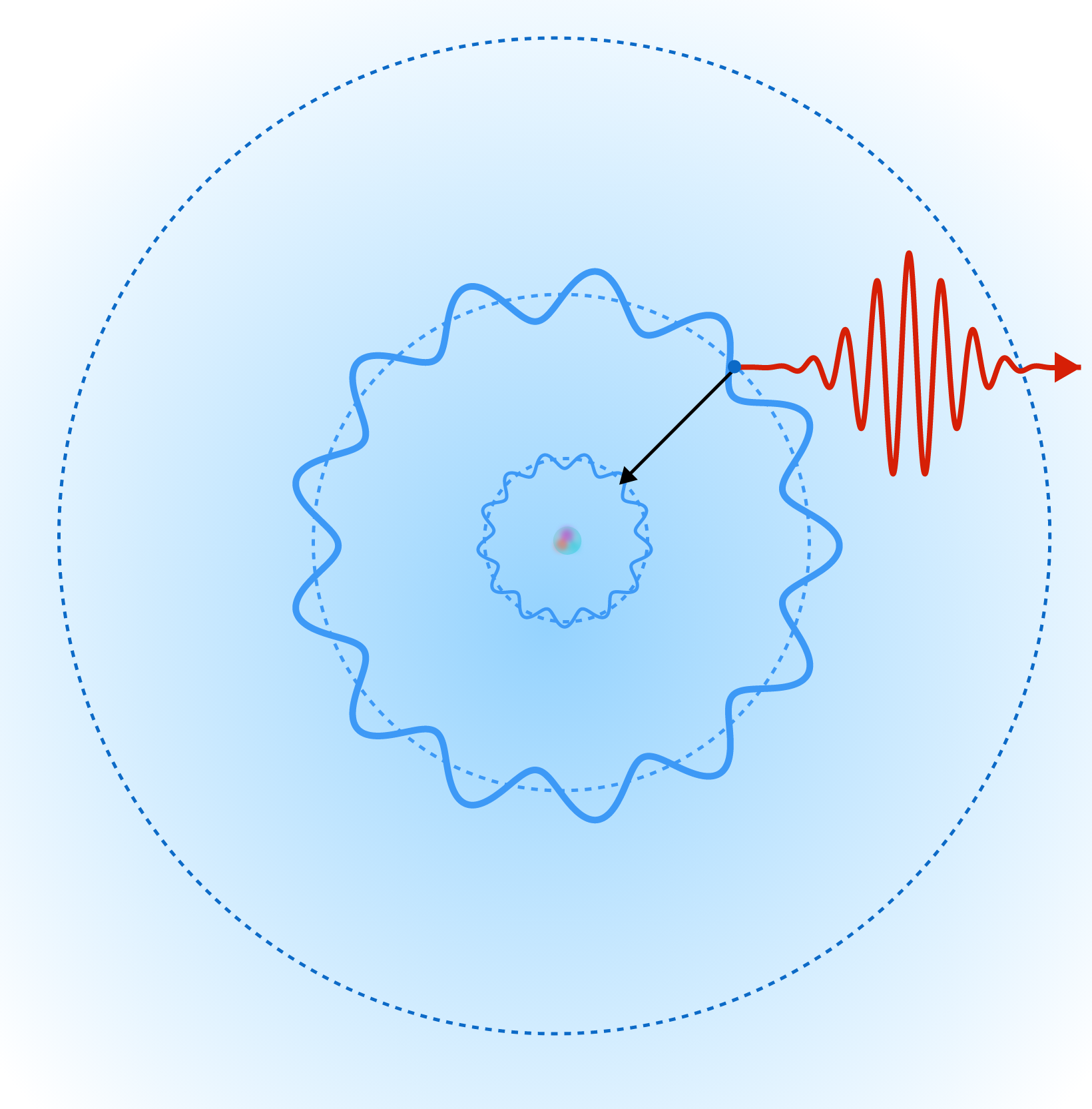Suppose an electron emits a photon when it transitions from an excited state of hydrogen with energy $-\SI{1.51}{\electronvolt}$ to another state with energy $-\SI{3.40}{\electronvolt}.$

What is this the frequency of the photon emitted?


Details and Assumptions:

• The energy of the photon is exactly equal to the amount of energy lost by the electron.
• In the last quiz, we introduced the fundamental relationship between photon energy and photon frequency, $E=hf,$ where Planck's constant $h=\SI{6.63e-34}{\joule\second}.$
• $\SI{1}{\electronvolt}=\SI{1.6e-19}{\joule}.$

# Atomic Spectra

The same absorption and emission process occurs high in Earth's atmosphere, and we see it as the aurora. Atmospheric gas atoms, directed by Earth's magnetic field, are excited by solar radiation. As electrons later transition into lower-energy states, light with very specific wavelengths is emitted by the nitrogen and oxygen, the most common gases in Earth's atmosphere.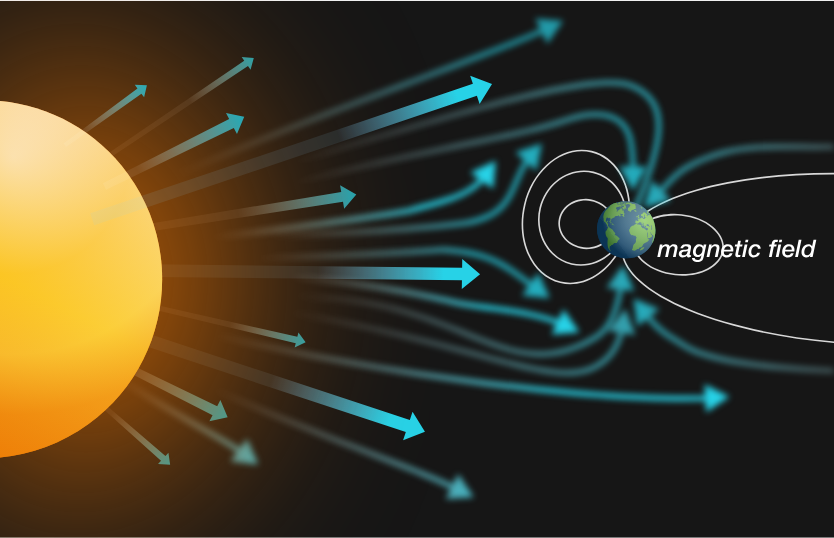If you measure the wavelength of light emitted by a green aurora, you would find its wavelength to be $\SI{557.7}{\nano\meter}.$ Below is a table with three electron states of oxygen. Can you find a transition between two of these states that produces the wavelength observed in an aurora? $\begin{array}{c|c} \hline \text{Orbital}& \text{Energy} \\ \hline \mathbf{A}& \SI{-13.62}{\electronvolt} \\ \hline \mathbf{B}& \SI{-10.99}{\electronvolt} \\ \hline \mathbf{C}& \SI{-8.77}{\electronvolt} \\ \hline \end{array}$

Details and Assumptions:

• $h=\SI{6.63e-34}{\joule\second}.$
• $c=\SI[per-mode=symbol]{3.0e8}{\meter\per\second}.$

# Atomic Spectra

We now have a more refined understanding of EM radiation: photons are emitted by electrons (and other charged particles) as they lose energy. In a hot gas, this leads to a characteristic line emission spectrum that astronomers use to identify the gas, no matter how far away the light is emitted. Knowledge of gas spectra is an indispensable tool for an astronomer studying the composition of objects across the universe, as it will be to us moving forward.

In this chapter, we have completed a crash course on matter in the universe, and the long-range forces important for understanding its structure. Astronomers and physicists assume that the basic properties of matter we observe on Earth can be extended beyond the edges of our galaxy, to the farthest objects whose light can be detected.Now, we are equipped to explore the brilliant work of astronomers through the ages and get a sense of our place in the universe.

# Atomic Spectra

×